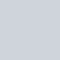# 題目出處

862 · Next Closest Time

medium

# 個人範例程式碼

``````class Solution:
"""
@param time: the given time
@return: the next closest time
"""
def next_closest_time(self, time: str) -> str:
digits = set(time)
hr, m = int(time[0:2]), int(time[3:5])
current = hr*60+m
# 0 - 1440
for i in range(1, 1441):
next_time = (current + i)%1440
next_time = self.change_format(next_time)
print(next_time)
if set(next_time) <= digits:
return next_time

def change_format(self, x):
hr, m = x//60, x%60
return f"{hr:02}:{m:02}"
``````

x

# Reference